# Lecture 14 data structures and algorithms

22. Nov 2013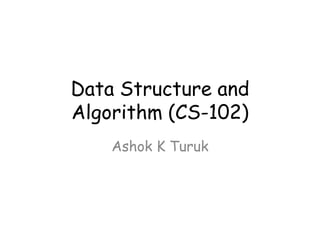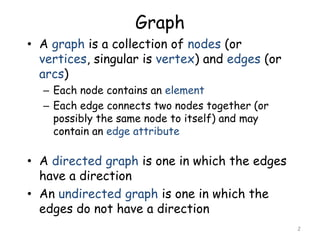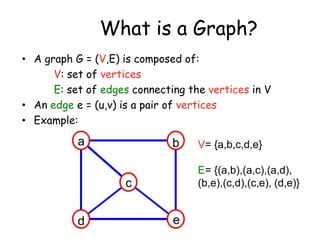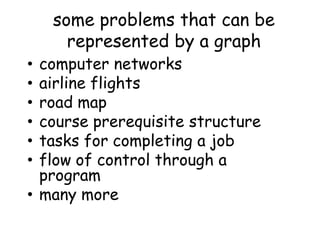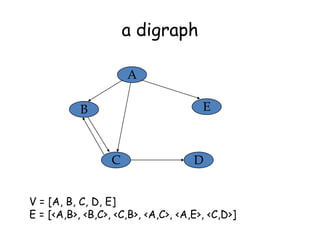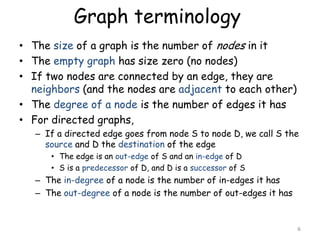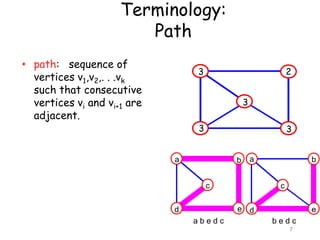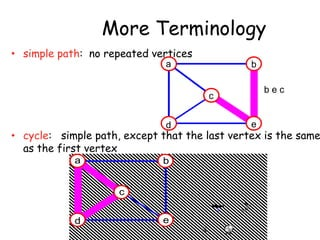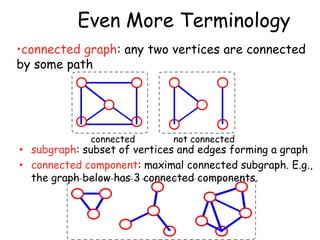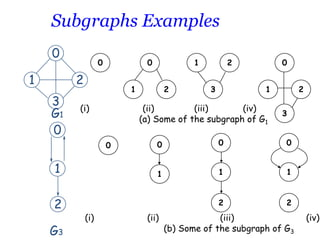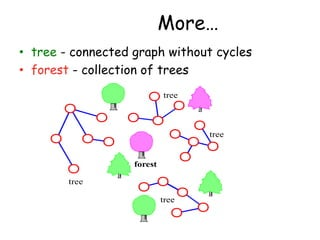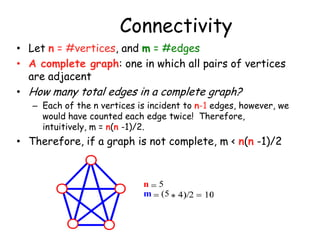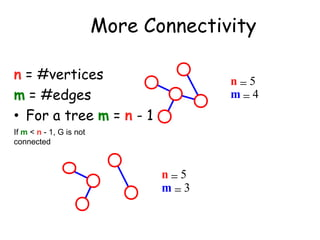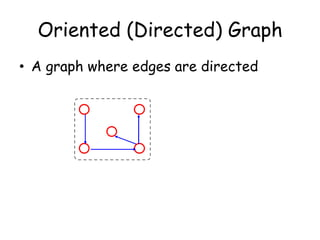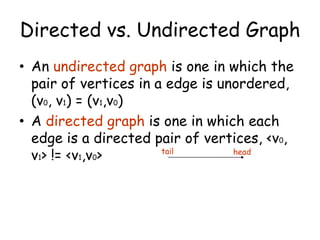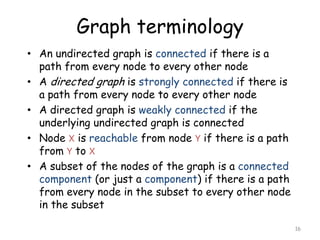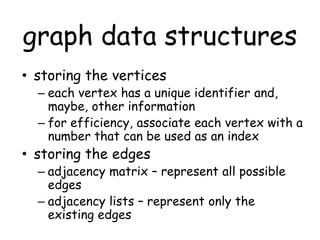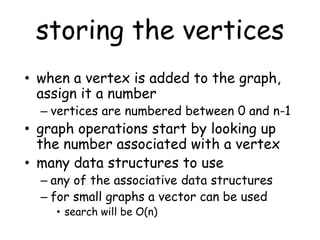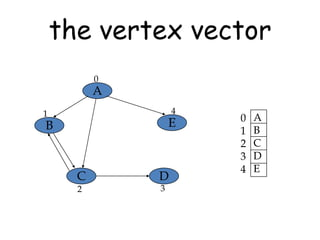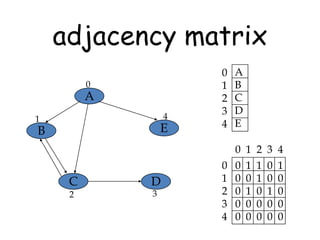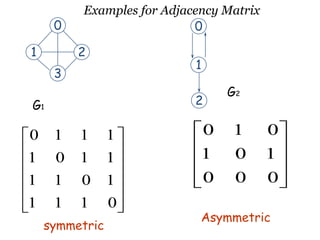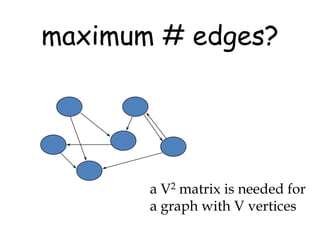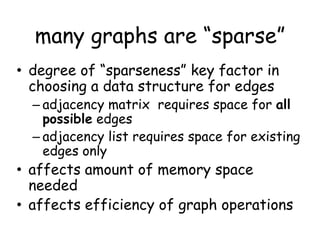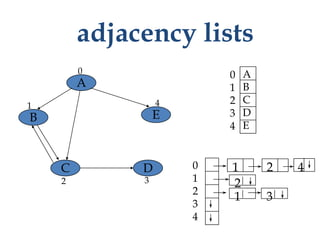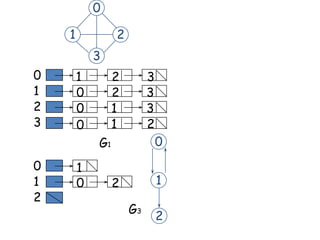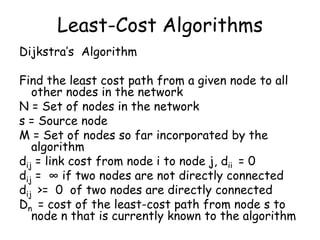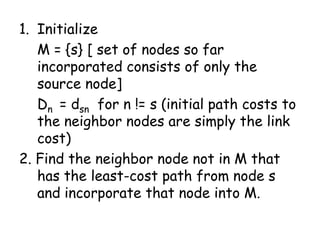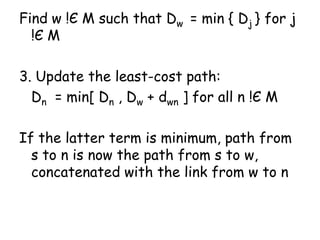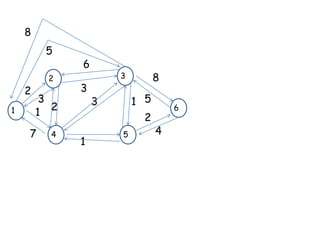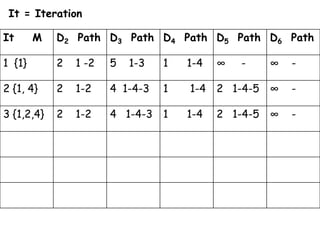1 von 30

### Lecture 14 data structures and algorithms

• 1. Data Structure and Algorithm (CS-102) Ashok K Turuk
• 2. Graph • A graph is a collection of nodes (or vertices, singular is vertex) and edges (or arcs) – Each node contains an element – Each edge connects two nodes together (or possibly the same node to itself) and may contain an edge attribute • A directed graph is one in which the edges have a direction • An undirected graph is one in which the edges do not have a direction 2
• 3. What is a Graph? • A graph G = (V,E) is composed of: V: set of vertices E: set of edges connecting the vertices in V • An edge e = (u,v) is a pair of vertices • Example: a b E= {(a,b),(a,c),(a,d), (b,e),(c,d),(c,e), (d,e)} c d V= {a,b,c,d,e} e
• 4. some problems that can be represented by a graph computer networks airline flights road map course prerequisite structure tasks for completing a job flow of control through a program • many more • • • • • •
• 5. a digraph A E B C D V = [A, B, C, D, E] E = [<A,B>, <B,C>, <C,B>, <A,C>, <A,E>, <C,D>]
• 6. Graph terminology • The size of a graph is the number of nodes in it • The empty graph has size zero (no nodes) • If two nodes are connected by an edge, they are neighbors (and the nodes are adjacent to each other) • The degree of a node is the number of edges it has • For directed graphs, – If a directed edge goes from node S to node D, we call S the source and D the destination of the edge • The edge is an out-edge of S and an in-edge of D • S is a predecessor of D, and D is a successor of S – The in-degree of a node is the number of in-edges it has – The out-degree of a node is the number of out-edges it has 6
• 7. Terminology: Path • path: sequence of vertices v1,v2,. . .vk such that consecutive vertices vi and vi+1 are adjacent. 3 2 3 3 3 a b a c b c e d d abedc e bedc 7
• 8. More Terminology • simple path: no repeated vertices a b bec c e d • cycle: simple path, except that the last vertex is the same as the first vertex a b acda c d e
• 9. Even More Terminology •connected graph: any two vertices are connected by some path connected not connected • subgraph: subset of vertices and edges forming a graph • connected component: maximal connected subgraph. E.g., the graph below has 3 connected components.
• 10. Subgraphs Examples 0 1 0 0 2 3 G1 0 1 (i) 3 0 1 2 3 0 0 1 1 2 (i) 0 1 1 G3 2 2 (ii) (iii) (iv) (a) Some of the subgraph of G1 0 2 1 2 (ii) (iii) (b) Some of the subgraph of G3 (iv)
• 11. More… • tree - connected graph without cycles • forest - collection of trees tree tree forest tree tree
• 12. Connectivity • Let n = #vertices, and m = #edges • A complete graph: one in which all pairs of vertices are adjacent • How many total edges in a complete graph? – Each of the n vertices is incident to n-1 edges, however, we would have counted each edge twice! Therefore, intuitively, m = n(n -1)/2. • Therefore, if a graph is not complete, m < n(n -1)/2 n 5 m (5
• 13. More Connectivity n = #vertices m = #edges • For a tree m = n - 1 n 5 m 4 If m < n - 1, G is not connected n 5 m 3
• 14. Oriented (Directed) Graph • A graph where edges are directed
• 15. Directed vs. Undirected Graph • An undirected graph is one in which the pair of vertices in a edge is unordered, (v0, v1) = (v1,v0) • A directed graph is one in which each edge is a directed pair of vertices, <v0, tail head v1> != <v1,v0>
• 16. Graph terminology • An undirected graph is connected if there is a path from every node to every other node • A directed graph is strongly connected if there is a path from every node to every other node • A directed graph is weakly connected if the underlying undirected graph is connected • Node X is reachable from node Y if there is a path from Y to X • A subset of the nodes of the graph is a connected component (or just a component) if there is a path from every node in the subset to every other node in the subset 16
• 17. graph data structures • storing the vertices – each vertex has a unique identifier and, maybe, other information – for efficiency, associate each vertex with a number that can be used as an index • storing the edges – adjacency matrix – represent all possible edges – adjacency lists – represent only the existing edges
• 18. storing the vertices • when a vertex is added to the graph, assign it a number – vertices are numbered between 0 and n-1 • graph operations start by looking up the number associated with a vertex • many data structures to use – any of the associative data structures – for small graphs a vector can be used • search will be O(n)
• 20. adjacency matrix 0 A 4 1 E B 0 1 2 3 4 A B C D E 0 1 2 3 4 C 2 D 3 0 1 2 3 4 0 0 0 0 0 1 0 1 0 0 1 1 0 0 0 0 0 1 0 0 1 0 0 0 0
• 21. 0 1 Examples for Adjacency Matrix 0 2 1 3 2 G1 G2 0 1 1 1 0 1 0 1 1 0 1 1 0 1 1 1 0 1 0 0 0 1 1 1 0 symmetric Asymmetric
• 22. maximum # edges? a V2 matrix is needed for a graph with V vertices
• 23. many graphs are “sparse” • degree of “sparseness” key factor in choosing a data structure for edges – adjacency matrix requires space for all possible edges – adjacency list requires space for existing edges only • affects amount of memory space needed • affects efficiency of graph operations
• 26. Least-Cost Algorithms Dijkstra’s Algorithm Find the least cost path from a given node to all other nodes in the network N = Set of nodes in the network s = Source node M = Set of nodes so far incorporated by the algorithm dij = link cost from node i to node j, dii = 0 dij = ∞ if two nodes are not directly connected dij >= 0 of two nodes are directly connected Dn = cost of the least-cost path from node s to node n that is currently known to the algorithm
• 27. 1. Initialize M = {s} [ set of nodes so far incorporated consists of only the source node] Dn = dsn for n != s (initial path costs to the neighbor nodes are simply the link cost) 2. Find the neighbor node not in M that has the least-cost path from node s and incorporate that node into M.
• 28. Find w !Є M such that Dw = min { Dj } for j !Є M 3. Update the least-cost path: Dn = min[ Dn , Dw + dwn ] for all n !Є M If the latter term is minimum, path from s to n is now the path from s to w, concatenated with the link from w to n
• 30. It = Iteration It M D2 Path D3 Path D4 Path D5 Path D6 Path 1 {1} 2 1 -2 5 2 {1, 4} 2 1-2 3 {1,2,4} 2 1-2 1-3 1 1-4 ∞ 4 1-4-3 1 1-4 4 1-4-3 1 1-4 - ∞ - 2 1-4-5 ∞ - 2 1-4-5 ∞ -